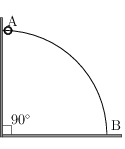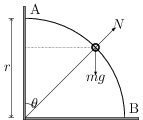# Circular Motion (Uniform and Non-Uniform)

## Problems from IIT JEE

Problem (IIT JEE 2011):A wire, which passes through the hole in a small bead, is bent in the form of a quarter of a circle. The wire is fixed vertically on ground as shown in the figure. The bead is released from near the top of the wire and it slides along the wire without friction. As the bead moves from A to B, the force it applies on the wire is,

1. always radially outwards.
2. always radially inwards.
3. radially outwards initially and radially inwards later.
4. radially inwards initially and radially outwards later.

Solution:Let $v$ be speed of the bead when it makes an angle $\theta$ with the vertical direction (see figure). The forces acting on the bead are its weight $mg$ and normal reaction $N$. Since all the forces acting on the bead are conservative, the mechanical energy of the bead is conserved i.e., \begin{align} \label{nxb:eqn:1} mgr(1-\cos\theta)=\tfrac{1}{2}mv^2. \end{align} The radially inward components of force provide centripetal acceleration to the bead i.e., \begin{align} \label{nxb:eqn:2} mg\cos\theta-N={mv^2}/{r}. \end{align} Eliminate $v^2$ from above equations to get, \begin{align} \label{nxb:eqn:3} N=mg(3\cos\theta-2). \end{align} From this equation, normal reaction $N$ is positive (radially outward) if $\theta < \cos^{-1}({2}/{3})$ and negative (radially inward) if $\theta > \cos^{-1}({2}/{3})$. By Newton's third law, force applied by the bead on the wire is $N^\prime=-N$, which is radially inwards initially and radially outwards later.